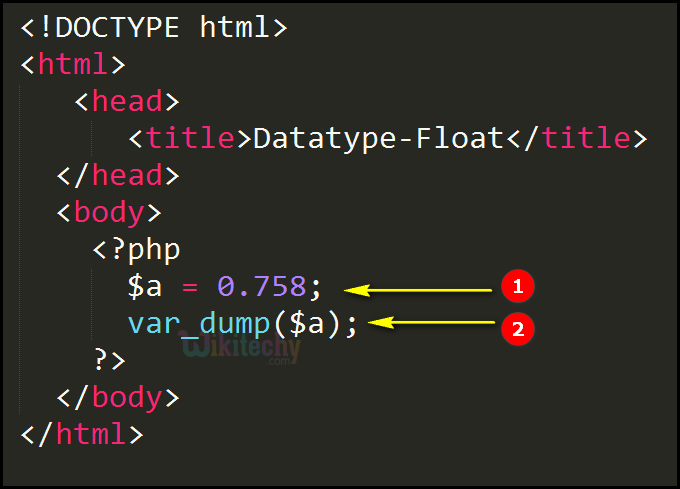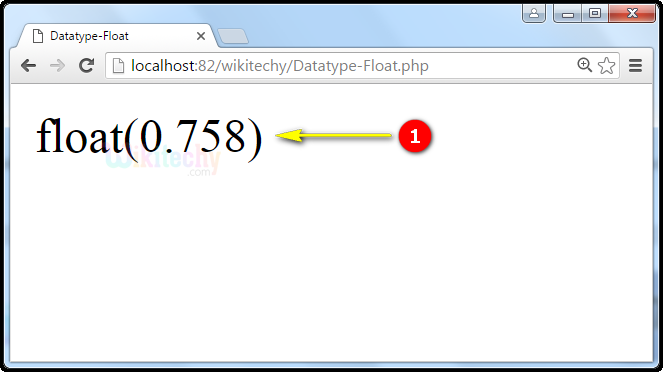# php tutorial - PHP Datatypes Float - php programming - learn php - php code - php script

• A floating point number is a number with a decimal point or a number in exponential form.
• Floating point numbers have limited precision.

## Syntax Explanation :

• Here \$x is a float.
• \$x is a float. The PHP var_dump() function returns the data type as float with its float value.

## php for beginners Code Explanation :1. In PHP, \$a = 0.758 is a float variable that is holds the integer value =0.758.
2. In PHP, var_dump() this function returns the data type as float with its float value as 0.758.

## php tutorials Sample Output :1. var_dump() function returns the float data type with its float value as 0.758 i.e., float(0.758).